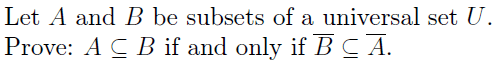# Logical Proofs Regarding Sets and Subsets

enkrypt0r

## Homework Statement

The following is all the information needed:## Homework Equations

There are, of course, all the basic rules of logic and set identities to be considered.

## The Attempt at a Solution

Not really sure how to attempt this one, to be honest. I know that (A ⊆ B) can be written with a quantifier and is equivalent to ∀x[xϵA ⇒ xϵB] but I'm really not sure how to apply this information properly.

Thanks, guys. :)

To prove $\overline{B}\subset\overline{A}$ start with "if x is a member of $\overline{B}$ then x is not in B. Since, by hypothesis, $A\subset B$ x is not in A. ...